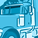QlikView App Dev

Discussion Board for collaboration related to QlikView App Development.

Announcements
QlikWorld 2022, LIVE in Denver CO., May 16-19, 2022. REGISTER NOW TO RECEIVE EARLY BIRD PRICING
cancel
Showing results for
Did you mean:Contributor II

Help: Count and sum confusion

Hi Guys,

I facing issue in taking count of state.

Please find below expression used in pivote table...

(sum({<Month={\$(vMaxMonth)}>}SalesNetValue)-sum({<Month={\$(VPrevMonth)}>}SalesNetValue))

/

sum({<Month={\$(VPrevMonth)}>}SalesNetValue)

Query: I want display count of states having salesnetvalue (using above expression) below zero.

1 Solution

Accepted SolutionsMVP

Your above expression is not returning a sales net value. It's calculating a change percentage of sales net value comparing two months'  aggregated values.

But using this expression and assuming you have a field called State, try an expression search like

Count(

{<

State = {"=

(sum({<Month={\$(vMaxMonth)}>}SalesNetValue)-sum({<Month={\$(VPrevMonth)}>}SalesNetValue))

/

sum({<Month={\$(VPrevMonth)}>}SalesNetValue)

<0"}

>}

DISTINCT State)

4 RepliesMVP

Your above expression is not returning a sales net value. It's calculating a change percentage of sales net value comparing two months'  aggregated values.

But using this expression and assuming you have a field called State, try an expression search like

Count(

{<

State = {"=

(sum({<Month={\$(vMaxMonth)}>}SalesNetValue)-sum({<Month={\$(VPrevMonth)}>}SalesNetValue))

/

sum({<Month={\$(VPrevMonth)}>}SalesNetValue)

<0"}

>}

DISTINCT State)Contributor II
Author

Hi Stefan,

I am Using below expression for  Pareto Classes.

Now I want to show count of States falling under each class.

Aggr(

If(Rangesum(Above(Sum({1} SalesNetValue)/Sum({1} total SalesNetValue),1,RowNo()))<0.8, 'A',

If(Rangesum(Above(Sum({1} SalesNetValue)/Sum({1} total SalesNetValue),1,RowNo()))<0.9, 'B',

'C')),

(StateCode,(=Sum({1} SalesNetValue),Desc))

)MVP

Try the Aggr() function as calculated dimension in a chart and as expression

=Count(Distinct StateCode)Contributor II
Author

Hi Stefan,

It is working fine if I take count for State as in Aggr I have used statecode...

but if I use statecode and distributor code in aggr and then use count of statecode and distributor code then values for statecode are correct but for distributor code it is giving wrong values....Community Browser